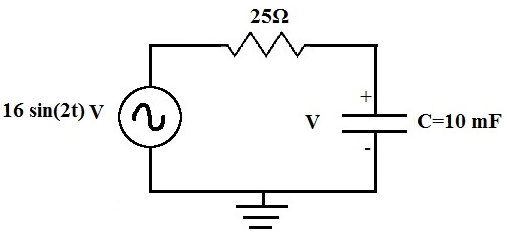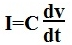﻿ What is a First Order Differential Circuit?

# What is a First Order Differential Circuit?A First Order Differential Circuit (or, just simply, first order circuit) is a circuit with one energy storage element, a capacitor or inductor. It is a called a first order differential circuit because in order to solve for the capacitor's or inductor's voltage or current, a first order differential equation must be computed to find the result.

Shown above is a circuit where the first order differential equation of the capacitor must be computed in order to find the voltage of the capacitor. The capacitor is the energy storage element of the circuit. In order to solve for its voltage, Kirchhoff's current Law (KCL) is applied to the circuit. Since a capacitor's current is equal to:The only way to solve for the voltage is to compute this differential term dv/dt which is of the first order.

Related Resources